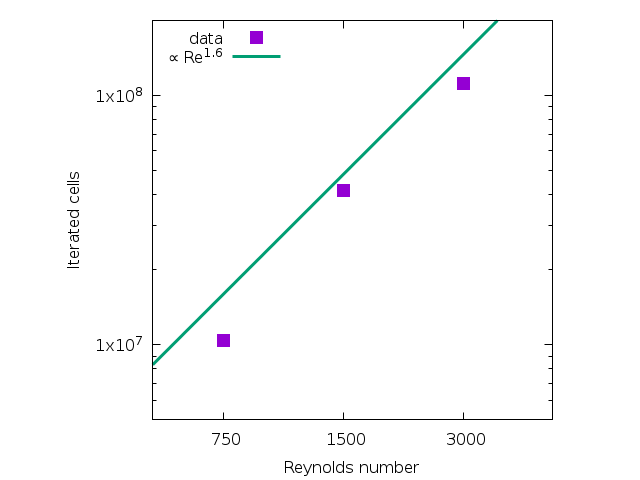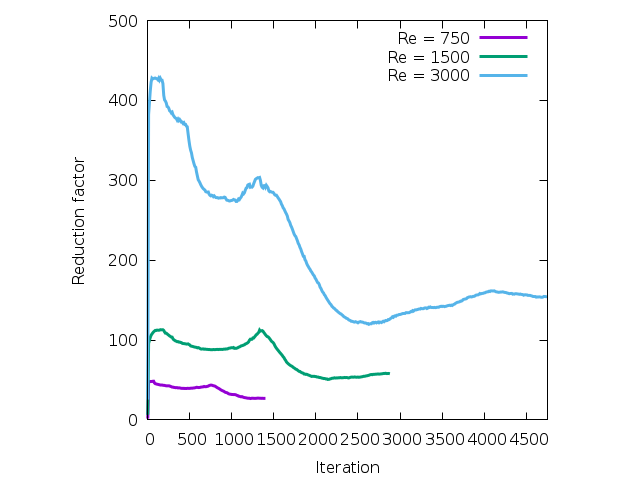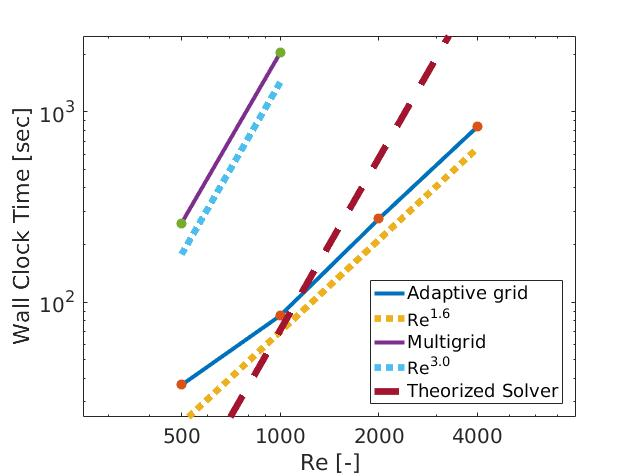# sandbox/Antoonvh/lamb-dipole.cThe dipolar vortex induced by this airplane may collide with the underlying surface. Image courtesy via The New York Times.

# The Collision of a Dipolar Vortex with a Wall

Inspired by the work of Orlandi (1990), we study the collision of a dipolar vortex with a no-slip wall. We consider a vortex structure inside a circular atmosphere with radius R thats propagates through a fluid with a velocity (U).

The vortex-wall collision dynamics are goverend by the interaction of the primary and secondary vorticity. The latter is the vorticity that orginates from the boundary layer at the no-slip wall due to the dipole-induced flow. The layer owes its existence to the fluid’s viscosity (\nu). With the system parameters \{R,U,\nu\}, a dimensionless group (\mathrm{Re}) can be identified,

\displaystyle \mathrm{Re} = \frac{U R}{\nu},

that is known as the Reynoldsnumber. On this page we study the effect of the Reynolds number on the evolution of the flow.

## The numerical model

We solve the incompressible Navier-Stokes equations.

#include "navier-stokes/centered.h"
#include "view.h"

u.t[left] = dirichlet (0.);

int maxlevel = 9;
double t_end = 12, vis;
long long unsigned int it;
FILE * fp;
char str;

A square box with size 15R\times15R is chosen and a momentum-conserving refinement attribute is used. The Reynolds number is directly controlled by the viscosity. We choose it such that \mathrm{Re}= \{750,\ 1500,\ 3000\}. For the scaling of the maximum refinement level, we follow Clerx en Van Heijst (2017).

int main() {
fp = fopen ("iteratedcells", "w");
L0 = 15.;
init_grid (1 << 8);
foreach_dimension()
u.x.refine = refine_linear;
vis = 1./750.;
run();
for (int j = 0; j < 2; j++) {
vis /= 2.;
maxlevel++;
run();
}
}

## The initial dipolar vortex

The flow is initialized with the Lamb-Chaplygin dipolar vortex (Meleshko and Van Heijst, 1994) vortex propagating towards the left-hand-side wall according to the stream function \psi,

\displaystyle \psi(r<R,\theta) = \frac{-2 U J_{1}(kr)}{kJ_{0}(k)}sin(\theta), and

\displaystyle \psi(r \geq R,\theta) = U\left(\frac{R^2}{r}+r\right)sin(\theta),

in spherical coordinates (r,\theta) where r is the distance from the dipole centre and \theta represents the angular coordinate.

We use macros for a cylindrical voordinate system:

#define RAD (pow(pow((x - xo), 2)+(pow((y - yo), 2)), 0.5))

event init (t = 0) {
sprintf (str, "Re = %g", 1./vis);
scalar psi[];
double xo = 4.7, yo = 7.6, k = 3.8317;
it = 0;
const face vector muc[] = {vis, vis};
mu = muc;
refine (RAD < 2.0 && level <= 9);
refine (RAD < 1.0 && level <= 10);
foreach()
boundary ({psi});
foreach() {
u.x[] = -((psi[0, 1] - psi[0, -1])/(2*Delta));
u.y[] = (psi[1, 0] - psi[-1, 0])/(2*Delta);
}
boundary (all);
}

The grid is adapted based on the wavelet-based estimated error with regards to the discretized representation of the velocity-component fields. Read here for a narrative on the used method.

event adapt (i++)
adapt_wavelet ((scalar *){u}, (double []){0.01, 0.01}, maxlevel);

## Results

The behaviour of the vorticty structures is visuzalized for all Reynolds numbers along with the grid evolution.

event bviewer (t = 1.; t += 0.075) {
scalar omega[];
vorticity (u, omega);
clear();
view (fov = 10, tx = 0.001, ty = -0.5, psi = 0.0001,
width = 700, height = 470, samples = 2);
squares ("omega", map = cool_warm, min = -10., max = 10.);
mirror ({1,0})
cells();
draw_string (str, pos = 3, lw  = 3, size = 20);
save ("lamb.mp4");
clear();
}

Here is the movie:

Evolution of the vorticity fields and the used grids for the three experiments.

Apart from the rebound of the primary vorticity, it appears there is some Reynolds-number dependence of the vorticity structures in the boundary layer. For extra detail, we zoom-in on the collision itself.

event inzoom (t = 4.25; t += 0.025; t<= 7.5) {
scalar omega[];
vorticity (u, omega);
clear();
view (fov = 3.5, tx = 0.4, ty = -0.06, psi = -pi/2.,
width = 900, height = 500, samples = 2);
squares("omega", map = cool_warm, min = -10., max = 10., linear = true);
cells();
draw_string (str, pos = 1, lw  = 3, size = 30);
save ("lambinz.mp4");
}

Here is the resulting movie that is rotated for better compatibility with your screen’s aspect ratio:

A slower movie of the vorticity structures in the boundary layer for the three experiments

## The fractal dimension of the numerical problem

It would be interesting to find out what dynamics are retrieved in the limit of a vanishing viscosity. However, that would entail using higher resolution grids and would require more computational resources. Therefore, we study the number of iterated cells over the simulation runs.

event iterated_cells (i++) {
foreach()
it++;
}

event end (t = t_end)
fprintf (fp,"%g\t%llu\n", 1./vis, it);

We plot our results:

set terminal pngcairo enhanced font 'Times-italic,12'
set xr [500:5000]
set yr [5E6:2E8]
set xlabel 'Reynolds number'
set ylabel 'Iterated cells'
set xtics (0, 750, 1500 ,3000)
set logscale y
set logscale x
set size square
set key top left
plot 'iteratedcells' u 1:2 w p ps 2 pt 5 t 'data' , \
400*x**1.6 lw 3 t '{/Symbol \265} Re^{1.6}'(script)

When employing naive, equidistant gridding, it is expected that number of total iterated cells scales with the third power of the scale separation. This is because there are two dimensions for space, and one for time (CFL condition). However, when using grid adaptivity, the numerical requirements may inherit some of the properties of the physical system.

Also we diagnose and plot the evolution of the reduction factor with regards to the number of used grid cells compared to an equidistant grid at the maximum resolution.

event frac (i += 10) {
char fname;
sprintf (fname, "cells%g", 1./vis);
static FILE * fpc = fopen (fname, "w");
int n = 0;
foreach()
n++;
fprintf (fpc, "%d\t%g\t%d\t%g\n", i, t, n, (double)(1<<(maxlevel*dimension))/(double)n);
fflush (fpc);
}
set xr [0:4750]
set xtics auto
set yr [0:500]
set xlabel 'Iteration'
set ylabel 'Reduction factor'
unset logscale y
unset logscale x

set key top right
plot 'cells750' u 1:4 w l lw 3 t 'Re = 750' , \
'cells1500' u 1:4 w l lw 3 t 'Re = 1500' , \
'cells3000' u 1:4 w l lw 3 t 'Re = 3000'(script)

Appearently, with an increasing separation of scales, the reduction factor increases to very healthy values.

One may wonder how the costs of the simulations presented above scale with the Reynolds number. With an increasing Reynolds number the boundary layer at the no-slip wall becomes shallower and the maximum resolution should be increased. As such we can run the simulation above for various Reynoldsnumbers with the associated maximum level of refinement and log the wall-clock time. The resulting flows are shown here on youtube and the costs are plotted in the figure below.Scaling of the wall-clock time of the simulation runs for different Reynoldsnumbers. The results are presented for the adaptive grid and fixed-resolution and equidistant multigrid simulations. Furthermore, theorized results are included to represent a fixed-grid code that is 20 times faster than the Basilisk multigrid approach

The scaling of the costs observed for the simulations with the multigrid approach is exactly as expected. The number of grid cells scales quadratically with the spatial resolution and the timestep is inversly proportional to the mesh size (via the CFL criterion). This results in (2\ \text{space} +1\ \text{time} = 3\ \text{dimensions}) so that the costs scale according to: \mathrm{Re}^{3}. How the costs of adaptive-grid simulations scale with the Reynoldsnumber is not so obvious. Rather than trying to explain the scaling, the observed scaling may tell us something about the fractional dimension of the problem: It indicates that the maximum resolution requirement is not space filling.

An iteresting remark is that for this case and the chosen Reynolds numbers the adaptive grid solver is always more efficent than the static approach. However, there may exist more efficient codes in the world. We could assume the existence of a fixed-grid code that is 20 times faster than the basilisk multigrid-based solver (e.g using spectral differencing on a GPU or so). We can already anticipate its performance by simply shifting down the plotted line asociated with the multigrid. However, the scaling of such a fixed-grid approach will remain to the third power as it would not be able to profit from the fractal dimension of this physical system. As such, there will be a Reynolds number where the adaptive-grid approach will ‘out perform’ the theorized very fast fixed-grid code. In this example, \text{Re}=1000 would be the break-even point.

Orlandi, P.: 1990, Vortex dipole rebound from a wall, Phys. Fluids A 2, 1429–1436.

Meleshko, V.V. and Van Heijst, G.J.F.: 1994, On Chaplygin’s investigations of two-dimensional vortex structures in an inviscid fluid, J. Fluid Mech. 272, 157–182.

Clercx, H.J.H. & van Heijst, G.J.F. : 2017, Dissipation of coherent structures in confined two-dimensional turbulence, Physics of Fluids, 29(11):111103